# OOF2: The Manual

## 2.5. Meshes and SubProblems

The OOF2 Mesh object represents an actual finite element Mesh. It combines the geometry of a Skeleton with information about equations and boundary conditions, and it stores the values of Fields. In OOF2 version 2.1 and later, the Mesh can store sets of Field values, with a different time for each set.

Every Mesh is derived from a Skeleton. The Mesh elements have the same shape and position as the associated Skeleton elements. The Material assigned to each Mesh element is the Material of the dominant pixel category of its Skeleton element. A single Skeleton may give rise to more than one Mesh.

### 2.5.1. Elements and Nodes

Mesh elements are analogous to Skeleton elements, but they contain more information. In particular, Mesh elements can perform finite element interpolation by means of shape functions. The shape functions form a basis for the expansion of functions on the mesh, just as the series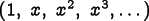forms a basis for the expansion of functions of a real number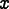. That is, any function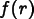, where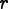is a point in the plane, can be approximated by the sum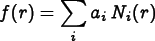(2.4)

where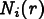is the ith shapefunction and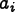is its coefficient. In finite elements, the shape functions are defined so that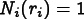(2.5)

for some position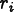, and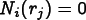(2.6)

for all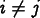. Therefore, the coefficients are simply given by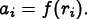(2.7)

These special positionsare called the nodes of the mesh. There are always nodes at the corners of an element, so the nodes of a Skeleton element always correspond to nodes of a Mesh element, but a Mesh element may have nodes along its edges or even in its interior.

The above equation means that a Field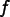can be determined by specifying its values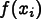only at the nodes. Thus we think of the Field values as living on the nodes of the mesh. The goal of a finite element solution is to find Field values at nodes.

Why did we bother to explain all this? OOF2 requires you to choose what kind of finite elements to use when creating a Mesh. Different types of elements differ in the shape functions that they provide and in the number and position of their nodes.

The polynomial order of the element shape functions determines the order of the interpolation within the element, and therefore affects the accuracy of the solution. (Fields computed with linear shape functions will be constrained to have constant gradients within elements, for example.) Because shape functions are 1 at one node and 0 at all others, the number of nodes along an element edge determines the order of the shape functions: an edge with nodes only at its endpoints must have linear shape functions, whereas an edge with nodes at its endpoints and one more in its interior must have quadratic shape functions.

There are actually three kinds of nodes: nodes used to store Field values (function nodes), nodes used to determine the element geometry (mapping nodes), and nodes used for both purposes. (Nodes determine element geometry by defining a mapping from a master element with a standard shape and position to the actual element. The mapping nodes of the master element are mapped to the nodes of the actual element, and all other points are mapped by using the mapping nodes' shape functions for interpolation. Non-linear mapping can produce elements with curved edges.) Elements with the same number of function and mapping nodes are called isoparametric. Elements with more function nodes than mapping nodes are called subparametric, and elements with more mapping nodes than function nodes are called superparametric.

Note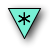Currently, although OOF2 allows high-order iso- and superparametric elements with non-linear mapping and curved element edges, it actually doesn't do anything useful with them. This is because Mesh elements are created from Skeleton elements, and Skeleton elements all have straight edges. The positions of interior nodes are determined by linear interpolation between the corner nodes, so the non-linear mapping from master elements to actual elements is effectively linear.

### 2.5.2. SubProblems

A Mesh may be divided into a number of SubProblems, which can be solved independently. SubProblems may differ from one another geographically, by including different Elements. They may also differ in which Fields and Equations are defined on them. For example, you might want to solve one set of equations on particulate inclusions and another set on the matrix in which they're embedded. Or in a time dependent problem, you might put the fast and slow degrees of freedom in separate subproblems and use different solution methods on each.

When a Mesh is created, a default SubProblem, called default, is created as well. The default SubProblem includes the entire Mesh. Users who don't need to use more complicated SubProblems can simply use the default, and can more or less forget that SubProblems exist at all. (Just think mesh when you read subproblem and you'll be fine.)

SubProblems are created in the Subproblem pane in the FE Mesh Task Page. The various kinds of SubProblems differ in their geographical extent. A MaterialSubProblem includes all Elements with a given Material type. A PixelGroupSubProblem includes all Elements which have a majority of their underlying pixels in a given PixelGroup. An EntireMeshSubProblem includes all Elements. There are also boolean SubProblems that are defined in terms of other existing SubProblems. The full set is documented in the SubProblemType class.

### 2.5.3. Fields and Time

Fields are the quantities, such as Temperature and Displacement, that OOF2 solves for. Fields have values at all points of the SubProblem or SubProblems on which they are defined. The Fields & Equations Task Page in OOF2 is used to change the status of Fields.

If a Field is defined on two or more geographically overlapping SubProblems on a Mesh, then the Field's values are the same for each SubProblem whereever the SubProblems overlap. That is, although Fields are defined on SubProblems, their values are actually only stored on the Mesh.

OOF2 can handle scalar Fields (such as Temperature), vector Fields (such as Displacement), and even tensor fields (such as liquid crystal order parameters).

Each Mesh also contains a time value. If a time dependent problem has been solved on a Mesh, the Mesh will store the values of the Fields at the starting time, the ending time, and possibly, if Outputs have been scheduled, at intermediate times as well, but there is always one time and one set of Fields that describe the current state of the Mesh. The current time can be changed either by initializing the Fields or by solving time dependent equations.

OOF2 sometimes needs to store the first time derivative of a Field. In this case, a new Field will automatically be created for it. The new Field's name is constructed by appending _t to the name of the original Field. For example, Displacement_t stores the first derivative of Displacement.

Storing all of the Field values at each time can use a lot of memory. OOF2 can cache the values either in RAM or in temporary files on a disk. The cache type for any Mesh can be changed in the Mesh Operations pane in the FE Mesh page. The default cache type, used for new Meshes, can be set in the OOF.Settings.Mesh_Defaults menu in the main window's menubar.

### 2.5.4. Fluxes

In OOF2 a Flux is a quantity analogous to stress in elasticity: it is generally some modulus times the derivative of a Field. Some examples are stress (elastic modulus times the strain, which is the symmetric gradient of the displacement), heat flux (thermal conductivity times the negative gradient of the temperature), and electrical polarization (permittivity times the negative gradient of the voltage). Properties define how Fields contribute to Fluxes. One flux may have contributions simultaneously from many different Properties and Fields.

Fluxes don't appear explicitly when setting up a problem, except when specifying Neumann or Generalized Force boundary conditions in the Boundary Condition page. After obtaining a solution, Fluxes may be computed for plots or analysis.

### 2.5.5. Removing The Third Dimension

OOF2 uses three dimensional material properties but only solves two dimensional problems. In elasticity, two standard ways of reducing three dimensional systems to two dimensions are plane strain and plane stress. Plane strain is implemented by constraining the out-of-plane components of the strain to be zero, and plane stress is (usually) implemented by using an effective in-plane elastic modulus.

OOF2 has to generalize the concepts of plane-stress and plane-strain because it deals with problems other than elasticity. Furthermore, since OOF2 allows couplings between Fields, more than one Field can contribute to a given Flux, and these couplings are not known in advance, it is not possible to compute effective in-plane moduli for generalized plane-stress problems.

OOF2's approach to the out-of-plane problem is to augment each Field with the out-of-plane components of its derivatives. For example, scalar Fields like Temperature become a pair of Fields, Temperature and Temperature_z, where Temperature_z is the z-derivative of the temperature. Displacement, which is a two-dimensional vector Field in the plane, is augmented with Displacement_z, which is the triplet of partial derivatives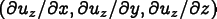, where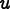is the (three dimensional) displacement field. Then the generalization of plane-strain simply sets these out-of-plane derivatives to zero and excludes them from the solution process. This is done for Fields that are marked as in-plane in the Fields & Equations task page. Note that Fields that are in-plane in one SubProblem must be in-plane in all SubProblems.

Plane-stress is generalized by augmenting the system of finite element equations by plane-flux equations that constrain the out-of-plane components of the Fluxes to be zero. This increases the size of the system of linear equations to be solved, but, unlike the method of defining effective in-plane moduli, works even if there are arbitrary couplings between fields.

### 2.5.6. Equations

Activating Equations in the Fields & Equations task page determines what physics problem OOF2 solves. There are (currently) two kinds of equations, divergence equations and plane-flux equations.

Equations are activated and deactivated on SubProblems. When we say that a SubProblem is solved, we really mean that its active Equations are solved in the portion of the Mesh that is included in the SubProblem.

Section 6.4.4 in Chapter 6 lists all of the predefined Equations in OOF2. Chapter 7 explains how to add new Equations.

#### Static Divergence Equations

Divergence equations are of the form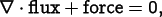(2.8)

where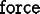is a generalized force. Examples are the force balance equation (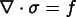, where f is an actual force) and the heat equation (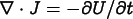, where the right hand side is a given rate of change of thermal energy density).

#### Nonstatic Equations

The general form of the time dependent version of the divergence equation is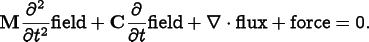(2.9)

For first order problems, e.g. the heat equation, the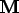term will be zero. For second order problems the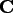term may be zero.

#### Plane Flux Equations

Plane flux equations implement OOF2's generalization of plane-stress. They simply state that the out-of-plane components of some flux are zero: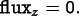(2.10)

Examples are the plane-stress equation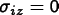,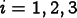, and the plane-heat-flux equation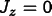.

#### Nonlinear Equations

OOF2 can solve nonlinear versions of Equations (2.8), (2.9), and (2.10), as long as the nonlinearity is confined to the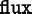andterms.

#### Parameters in Equations

The exact form of the Equations that OOF2 solves is determined by a number of things:

### 2.5.7. Boundary Conditions

OOF2 provides five kinds of boundary conditions, Dirichlet, Neumann, Floating, Generalized Force and Periodic. They can be applied only at boundaries that have been defined in the Skeleton from which the Mesh was derived. The Fields and Equations referred to in the boundary conditions need to be active.

• A Dirichlet boundary condition specifies the value of one component of a Field along a boundary.

• A Neumann boundary condition specifies the normal component of a Flux on a boundary.

• A Floating boundary condition is like a Dirichlet condition, except that the Field values along the boundary can vary by an unknown offset, which will be solved for. That is, if the condition specifies that the field values are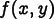, the actual values will be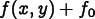where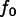is unknown, but has the same value at all points on the boundary.

• A Force boundary condition specifies the generalized force on each Node in the boundary. It is a discrete version of a Neumann condition, appropriate if you really have point sources of mechanical force or heat flux, for example.

• A Periodic boundary condition specifies that a component of a Field on the boundary has the same value as the Field on the boundary on the opposite side of the system. Periodic boundary conditions can only be applied to Meshes built from periodic Skeletons.

The Boundary Conditions Task Page contains the GUI for constructing and manipulating boundary conditions. The underlying commands are in the OOF.Mesh.Boundary_Conditions menu. The boundary condition objects themselves are in the BC class.

Boundary conditions may be explicitly enabled or disabled after they've been defined, using the button on the Boundary Conditions page. This allows you to switch back and forth easily to see the effects of different boundary conditions on a solution.

NoteIf you are using non-trivial SubProblems, be careful about boundary conditions on edges between the SubProblems. A future version of OOF2 will have tools to make it easy to apply the correct boundary conditions. But in version 2.1 if you solve for a field in one region, you can get unexpected field gradients in neighboring elements.

#### 2.5.7.1. Profiles

A Profile object describes the shape of a boundary condition. That is, a boundary condition fixes some quantity (a Field component, for example) on a boundary and the Profile of the boundary condition specifies how that quantity varies along the length of the boundary.

There are three kinds of Profiles, used by different kinds of boundary conditions. The graphical user interface takes care of selecting the right kind for you, but if you edit scripts, you need to use the correct type:

• Subclasses of ProfileX are Profiles that vary in position but not in time. They're used in Floating boundary conditions.

• Subclasses of ProfileXT are Profiles that can vary in time as well as position. They're used in Neumann and Force boundary conditions.

• Subclasses of ProfileXTd are also Profiles that can vary in time and position, but if they vary in time, the time derivative must be provided. They're used in Dirichlet boundary conditions.

### 2.5.8. Initial Conditions and Time

In time dependent problems, the initial values of each active Field must be set. In static linear problems, setting the initial value is optional, but a good choice of initial value can help the iterative solvers converge quickly. In static nonlinear problems, the choice of initial values can affect the solution.

If the active Equations include second time derivatives, initial values must also be provided for the Fields' first time derivatives.

Field initialization is a Mesh-wide operation, and is not specific to a particular SubProblem. When a Field is initialized, a value is assigned to it at all Nodes where the Field is defined.

The initial values of the Fields are set in two steps. In the first step, the initialization method for each Field is defined (OOF.Mesh.Set_Field_Initializer). Initializers can be constants or functions, or they can copy values from another Mesh. In the second step, all of the initializers for all of the defined Fields on the Mesh are applied simultaneously. This step can optionally also set the Mesh's current time. See OOF.Mesh.Apply_Field_Initializers and OOF.Mesh.Apply_Field_Initializers_at_Time.

Fields without initializers are unchanged when initializers are applied. If those Fields have never been solved or initialized, their values are undetermined, but are probably zero. It's a good idea to initialize all Fields.

### 2.5.9. Solvers

After Materials have been defined and assigned to pixels, a Mesh has been created, Fields have been defined and activated, equations have been activated and boundary conditions have been applied, the next step in solving a Mesh is to choose a solution method for each SubProblem. OOF2 lets you choose a solver in one of two ways:

• In basic mode you only need to set some general parameters. The specific algorithms that OOF2 will use are chosen automatically.
• In advanced mode you have to choose the specific algorithms and set all of their parameters.

Both solver modes let you choose a time stepping method. There are three kinds of time steppers:

• Static steppers don't actually take any time steps, they just solve for the instantaneous values of the Fields at the current time. If the Equations includeorterms, those terms will be ignored. OOF2 versions prior to 2.1.0 only included static steppers.

• Uniform steppers take time steps of a fixed size, which you have to specify.

• Adaptive steppers adjust the size of their time steps in order to keep the estimated error below a given tolerance. You have to specify the tolerance and a minimum allowable step size. Adaptive steppers have to do more computation than uniform steppers on each step, but can often achieve the same accuracy with fewer steps, and therefore are generally preferred.

All of the steppers have to solve a matrix equation at some point on each step. The matrix has one row for each Equation component being solved at every Node in the Mesh, and one column for each Field component at every Node. Therefore the matrices can be quite large. However, they are also generally sparse, meaning that most of its entries are zero. Large sparse matrix equations are best solved by iterative methods, which don't require the zeros to be stored explicitly, but which only provide approximate solutions. Direct matrix solvers, such as Gaussian elimination, may give more accurate solutions, but require a lot more storage and are not suitable for very large problems. In basic solver mode, you must choose either an iterative or direct matrix method. In advanced mode, you have to choose two matrix methods, for symmetric and asymmetric matrices. The choices include a number of different iterative techniques and a direct solver.

If the Materials have nonlinear Properties, either the matrix or the right hand side vector will depend on the Field values, and a nonlinear matrix solver must be selected. The nonlinear solvers use the linear solvers, so you still need to specify a linear matrix method. In basic solver mode, the nonlinear equations are detected and a nonlinear solver is chosen automatically. In advanced mode, you have to select one of OOF2's nonlinear solvers, Newton and Picard. Newton uses derivative information and generally converges faster than Picard, although it requires more computation per iteration. If the problem is linear, you should select None for the nonlinear solver. Picard and Newton will work on linear problems, but they'll do more work than necessary.

### 2.5.10. Solving

The Solver Page lets you solve static, quasi-static, and time dependent systems. Which one it does depends on the solvers that have been assigned to each SubProblem and on the setting of the end time with respect to the Mesh's current time:

• To compute a solution to a static problem, for which static solvers have been chosen for all active SubProblems, the end time set on the Solver Page must be equal to the current time, or empty.

• To compute the solution to a time dependent problem, adaptive or uniform solvers must assigned to the SubProblems, and the end time must be set to a time greater than the current time.

• A quasistatic problem is one in which the Fields are completely equilibrated at each time step. To compute a quasistatic solution with OOF2, assign static solvers to the SubProblems and set the end time to a time greater than the current time.

Time dependent and quasistatic subproblems can be solved together, by setting the end time greater than the start time, and assigning a static solver to some SubProblems and an adaptive or uniform solver to other SubProblems.

The starting time for a time dependent or quasistatic computation is determined by the initial conditions. The end time is set by the end time box in the Solver page. After solving, a solution may be continued by increasing the end time and solving again. As long as the Materials and Equations have not been changed, or the Fields have not been reinitialized, a continued calculation is indistinguishable from a calculation that used the new end time originally.

When multiple SubProblems are being solved, each is addressed in turn. Because the SubProblems may not be independent, the whole procedure is iterated until consistent answers are obtained. For time-dependent problems, this iteration takes place at every time step. For time-dependent problems with adapative solvers, the time step will be the smallest step chosen by all of the solvers.

### 2.5.11. Outputs and Analyses

After solving a Mesh, it's possible to view the resulting Fields and other quantities that depend on them. These quantities are generically called Outputs, and are discussed in Section 6.3. Outputs can be plotted in contour plots (both plain and filled) in the graphics window, queried at single points with the data viewer in the Mesh Info toolbox in the graphics window, or sent through various analysis routines in the Analysis and Boundary Analysis pages. The combination of an Output, the Operations performed on it, the Domain from which the data is taken, and the way in which the domain is sampled is called an Analyis.

Analyses can be scheduled to occur at particular times during a solution, by using the Scheduled Output page or the commands in the OOF.Mesh.Scheduled_Output menu. When Outputs are scheduled, the time stepper will adjust its steps so that it finishes a step at each output time. Any quantity that can be computed on the Analysis or Boundary Analysis pages can be used as a Scheduled Output. Operations such as updating the Graphics Window and saving the Mesh can also be scheduled.

To make it easier to reuse Analyses, they can be given names on the Analysis or Boundary Analysis pages. A Named Analysis can be retrieved and reused either on the page where it was defined, or as a Scheduled Output. A Named Analysis defined on one Mesh can be used on any Mesh on which its Output is computable and its Domain is defined.

### 2.5.12. Rebuilding Meshes

If a Skeleton is modified, such as by moving Nodes, refining Elements, defining or removing boundaries, or undoing or redoing a previous modification, any Meshes belonging to that Skeleton are declared to be out of sync, and most operations on the Meshes are forbidden until the Meshes have been rebuilt. The rebuilt Mesh will retain all of the SubProblems and boundary conditions of the old Mesh, and Field values will be preserved, although they may have to be interpolated onto the new positions of the Nodes.

### 2.5.13. Mesh Status

A brief description of the state of the current Mesh is displayed in the Info pane in the FEMesh page and in the Status pane of the Solver page. Clicking the button on the Solver page prints more information in the Message window.

The status messages are:

 This an extremely simplified discussion. Please see a real finite element text book for the details.

 OOF2 can handle tensor fields in the sense that the infrastructure for doing so is present. There are currently no tensor fields implemented and no material properties that use them.

 Scheduled Outputs should really be called something else, to avoid confusion with the Output classes, to which they are related but are not identical. We apologize.